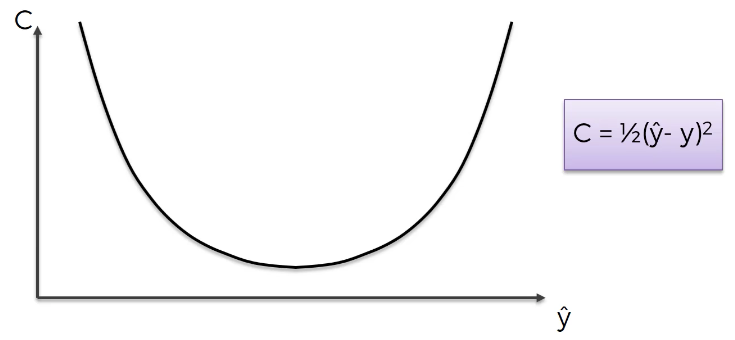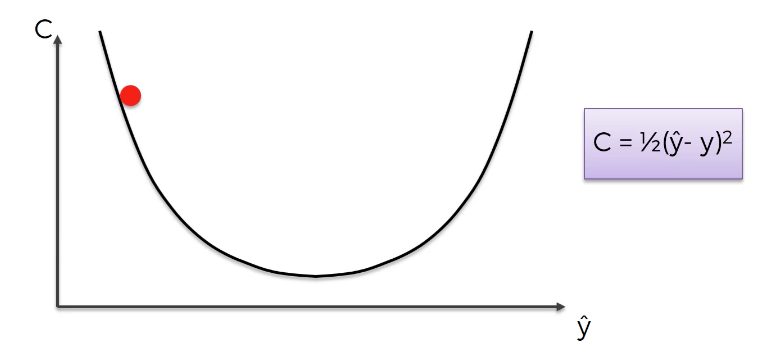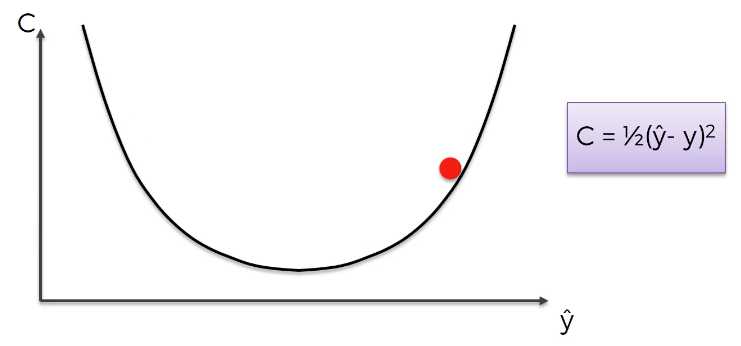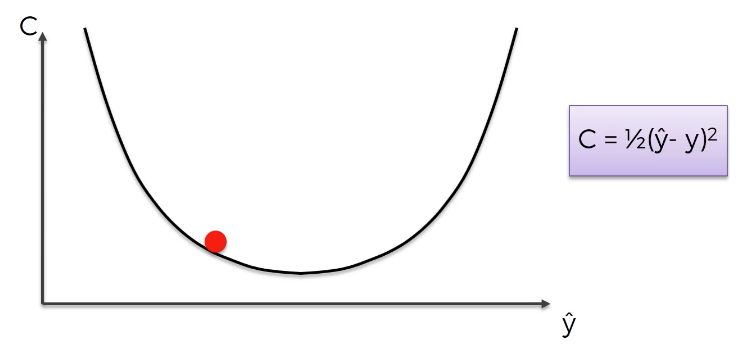# The Role of Gradient Descent in Deep Learning

Hey - Nick here! This page is a free excerpt from my \$199 course Python for Finance, which is 50% off for the next 50 students.

As a quick recap, in the last section of this deep learning course we discussed how neural networks are trained.

We briefly mentioned the term `gradient descent` in that tutorial. However, we did not dive into the meaning of gradient descent in any major detail.

This tutorial will provide an introduction to gradient descent and the role of gradient descent in deep learning.

## The Role of Gradient Descent in Deep Learning

Gradient descent is combined with another algorithm called `backpropogation` (which we'll explore in more detail later in this course) to change synapse weights at each neuron in a neural network when that neural network is initially trained.

More specifically, gradient descent is used to minimize the value of a neural network's cost function. There are a number of different ways this can be done. Two possible examples are below:

• By testing out a large random sample of synapse weights and choosing the weightings with the lowest cost function
• By testing every possible combination of weights and choosing the set with the lowest cost function (which is impossible to scale for obvious reasons)

While these methods are technically valid, they are far from optimal. This brute force approach is hampered by the `curse of dimensionality`.

The curse of dimensionality refers to the high computational cost involved with using brute force techniques to optimize high-dimensionality data.

As you might imagine, calculating different values for the cost function for randomly generated weights (or every possible weight) requires a lot of compute power - and this cost increases exponentially as the number of dimensions in the data set increases.

To solve this problem, the real way that gradient descent optimizes the cost function is by using differential calculus.

## Differential Calculus and Gradient Descent

Differential calculus is a fascinating field of mathematics whose primary use is calculating the slope of a line at specific points.

While the actual principles of differential calculus are outside of the scope of this course, it's sufficient to know that we can use differential calculus to `descend` down the slope of our cost function. This is where `gradient descent`'s name comes from.

Let's see this in action. To start, let's plot out the cost function that you were introduced to in the last section of this course:It is clear to the human eye where on this graph the cost function attains its lowest value. However, getting a neural network to modify its weights to achieve this cost function value is another problem entirely.

Fortunately, calculus does the trick. Let's start our cost function with an initial value on the left side of the graph, like this:Differential calculus allows us to determine that the slope of the cost function at this point is negative. Accordingly, the input value of the cost function (which is our predicted value) should be increased, which moves it to the right on this graph.Now the slope of the cost function at this point is positive. To decrease the cost function further, we must decrease its input value, which moves the function to the left:This process continues until the cost function is minimized. Here's a visual representation of what this looks like:Please note that this tutorial's goal was simply to provide a simple introduction to the basics of gradient descent. There are a number of factors related to gradient descent that you'll learn more about later, including:

• Choosing the proper cost function for your neural network
• Selecting how much to shift the weights in each iteration of a gradient descent algorithm (this is called the `learning rate`)

## Final Thoughts

This tutorial provided a brief, code-free introduction to the role of gradient descent in deep learning.

Here is a broad summary of what we discussed in this section:

• That gradient descent is combined with backpropagation to minimize the value of a neural networks' cost function during the training phase
• How the curse of dimensionality prevents us from using brute force techniques to optimize the value of a neural network's cost function
• Why differential calculus is used (instead of brute force methods) to optimize a cost function
• A visual interpretation of how the cost function is reduced in each iteration of a gradient descent algorithm